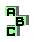POINT X(80)

 r604 incenter, Fuhrmann circle, inverse points r605 orthologic triangles, Fuhrmann triangle r606 excenters r767 isogonal points, incenter, circumcircle, inverse points r768 Euler line, Evans perspector r769 Evans perspector, incenter, cevian quotient r1039 Feuerbach point, pedal triangle, excenters, isogonal points r1118 circumcenter, cevian triangle, Gergonne point, perspective, incenter r1736 Feuerbach point, point X(65), isogonal points, concyclic points r1761 excircles r1763 incenter, point X(100), complement r1905 excenters, altimedial triangles, altitude feet r1947 incenter, Euler line, excenters, point X(100) r2011 intouch triangle, antimedial triangle, Euler line, incircle, Feuerbach point